# Spectral density, estimator of the

A function of the observed values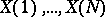of a discrete-time stationary stochastic process, used as an estimator of the spectral density. As an estimator of the spectral density one often uses quadratic forms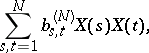where the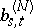are complex coefficients (depending on). It can be shown that the asymptotic behaviour as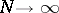of the first two moments of an estimator of the spectral density is satisfactory, in general, if one considers only the subclass of quadratic forms such that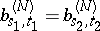when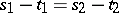. This enables one to restrict attention to estimators of the spectral density of the form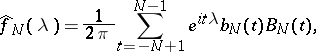where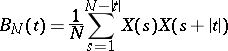is a sample estimator of the covariance function of the stationary processand theare suitably chosen weights. The estimator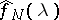can be written as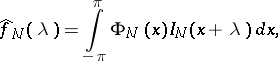where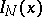is the periodogram and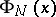is some continuous even function withof its Fourier coefficients specified: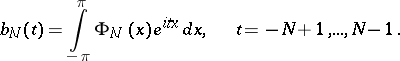The functionis called a spectral window; one usually considers spectral windows of the form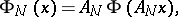whereis some continuous function on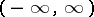such thatandas, but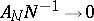. Similarly, one considers coefficientsof the form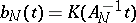and a function, called a lag window or covariance window. Under weak smoothness restrictions on the spectral density, or assuming that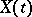is mixing, it is possible to prove that for a wide class of spectral or covariance windows the estimatoris asymptotically unbiased and consistent.

In the case of a multi-dimensional stochastic process, estimation of the elements of the matrix of spectral densities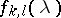proceeds in a similar way using the corresponding periodogram. Instead of an estimator of the spectral density in the form of a quadratic form in the observations, one often assumes that the spectral density depends in a particular way on a finite number of parameters, and then one seeks estimators based on the observations of the parameters involved in this expression for the spectral density (see Maximum-entropy spectral estimator; Spectral estimator, parametric).